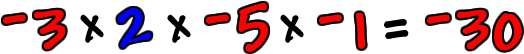So, for these, it's the same as before...

 1) Multiply the numbers your usual way 2) Deal with the signs

Here are a couple of examples: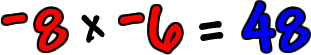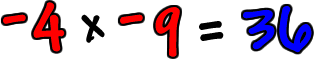Try it: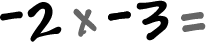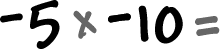OK, so what if we have more than two numbers to multiply?This is easy...  Same as before!  Just ignore the signs, multiply your usual way...  Then, deal with the weird sign thing!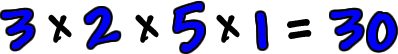Count up how many negative guys there are...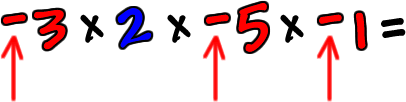There are three negative numbers.

 If there's an odd number of negatives, the answer is negative!

(Do you see why?  Think about it.  Pairs of negatives will turn positive, so, if you've got one negative guy left, the whole thing ends up being a positive times a negative.)